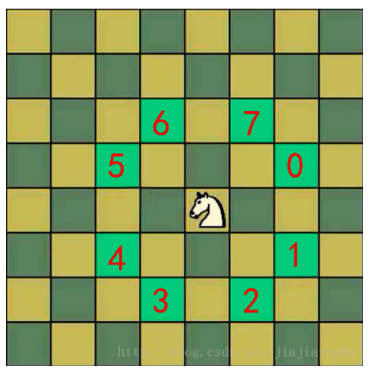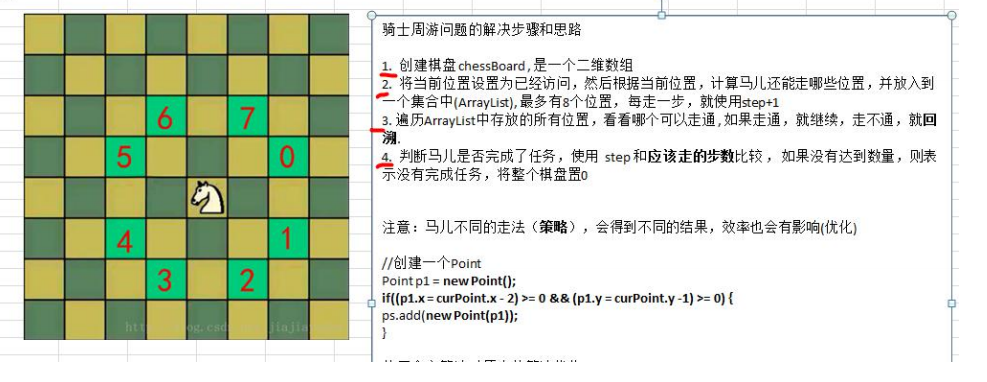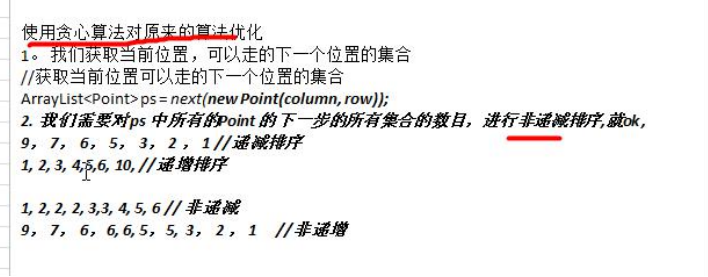5G时代下一个聚合的编程学习网

# 马踏棋盘算法介绍# 马踏棋盘算法分析# 代码实现

``````
import java.awt.Point;
import java.util.ArrayList;
import java.util.Comparator;

public class HorseChessboard {

private static int X; // 棋盘的列数
private static int Y; // 棋盘的行数
//创建一个数组，标记棋盘的各个位置是否被访问过
private static boolean visited[];
//使用一个属性，标记是否棋盘的所有位置都被访问
private static boolean finished; // 如果为true,表示成功

public static void main(String[] args) {
System.out.println("骑士周游算法，开始运行~~");
//测试骑士周游算法是否正确
X = 8;
Y = 8;
int row = 1; //马儿初始位置的行，从1开始编号
int column = 1; //马儿初始位置的列，从1开始编号
//创建棋盘
int[][] chessboard = new int[X][Y];
visited = new boolean[X * Y];//初始值都是false
//测试一下耗时
long start = System.currentTimeMillis();
traversalChessboard(chessboard, row - 1, column - 1, 1);
long end = System.currentTimeMillis();
System.out.println("共耗时: " + (end - start) + " 毫秒");

//输出棋盘的最后情况
for(int[] rows : chessboard) {
for(int step: rows) {
System.out.print(step + "	");
}
System.out.println();
}
}

/**
* 完成骑士周游问题的算法
* @param chessboard 棋盘
* @param row 马儿当前的位置的行 从0开始
* @param column 马儿当前的位置的列  从0开始
* @param step 是第几步 ,初始位置就是第1步
*/
public static void traversalChessboard(int[][] chessboard, int row, int column, int step) {
chessboard[row][column] = step;
//row = 4 X = 8 column = 4 = 4 * 8 + 4 = 36
visited[row * X + column] = true; //标记该位置已经访问
//获取当前位置可以走的下一个位置的集合
ArrayList<Point> ps = next(new Point(column, row));
//对ps进行排序,排序的规则就是对ps的所有的Point对象的下一步的位置的数目，进行非递减排序
sort(ps);
//遍历 ps
while(!ps.isEmpty()) {
Point p = ps.remove(0);//取出下一个可以走的位置
//判断该点是否已经访问过
if(!visited[p.y * X + p.x]) {//说明还没有访问过
traversalChessboard(chessboard, p.y, p.x, step + 1);
}
}
//判断马儿是否完成了任务，使用   step 和应该走的步数比较 ，
//如果没有达到数量，则表示没有完成任务，将整个棋盘置0
//说明: step < X * Y  成立的情况有两种
//1. 棋盘到目前位置,仍然没有走完
//2. 棋盘处于一个回溯过程
if(step < X * Y && !finished ) {
chessboard[row][column] = 0;
visited[row * X + column] = false;
} else {
finished = true;
}

}

/**
* 功能： 根据当前位置(Point对象)，计算马儿还能走哪些位置(Point)，并放入到一个集合中(ArrayList), 最多有8个位置
* @param curPoint
* @return
*/
public static ArrayList<Point> next(Point curPoint) {
//创建一个ArrayList
ArrayList<Point> ps = new ArrayList<Point>();
//创建一个Point
Point p1 = new Point();
//表示马儿可以走5这个位置
if((p1.x = curPoint.x - 2) >= 0 && (p1.y = curPoint.y -1) >= 0) {
}
//判断马儿可以走6这个位置
if((p1.x = curPoint.x - 1) >=0 && (p1.y=curPoint.y-2)>=0) {
}
//判断马儿可以走7这个位置
if ((p1.x = curPoint.x + 1) < X && (p1.y = curPoint.y - 2) >= 0) {
}
//判断马儿可以走0这个位置
if ((p1.x = curPoint.x + 2) < X && (p1.y = curPoint.y - 1) >= 0) {
}
//判断马儿可以走1这个位置
if ((p1.x = curPoint.x + 2) < X && (p1.y = curPoint.y + 1) < Y) {
}
//判断马儿可以走2这个位置
if ((p1.x = curPoint.x + 1) < X && (p1.y = curPoint.y + 2) < Y) {
}
//判断马儿可以走3这个位置
if ((p1.x = curPoint.x - 1) >= 0 && (p1.y = curPoint.y + 2) < Y) {
}
//判断马儿可以走4这个位置
if ((p1.x = curPoint.x - 2) >= 0 && (p1.y = curPoint.y + 1) < Y) {
}
return ps;
}

//根据当前这个一步的所有的下一步的选择位置，进行非递减排序, 减少回溯的次数
public static void sort(ArrayList<Point> ps) {
ps.sort(new Comparator<Point>() {

@Override
public int compare(Point o1, Point o2) {
// TODO Auto-generated method stub
//获取到o1的下一步的所有位置个数
int count1 = next(o1).size();
//获取到o2的下一步的所有位置个数
int count2 = next(o2).size();
if(count1 < count2) {
return -1;
} else if (count1 == count2) {
return 0;
} else {
return 1;
}
}

});
}
}
``````